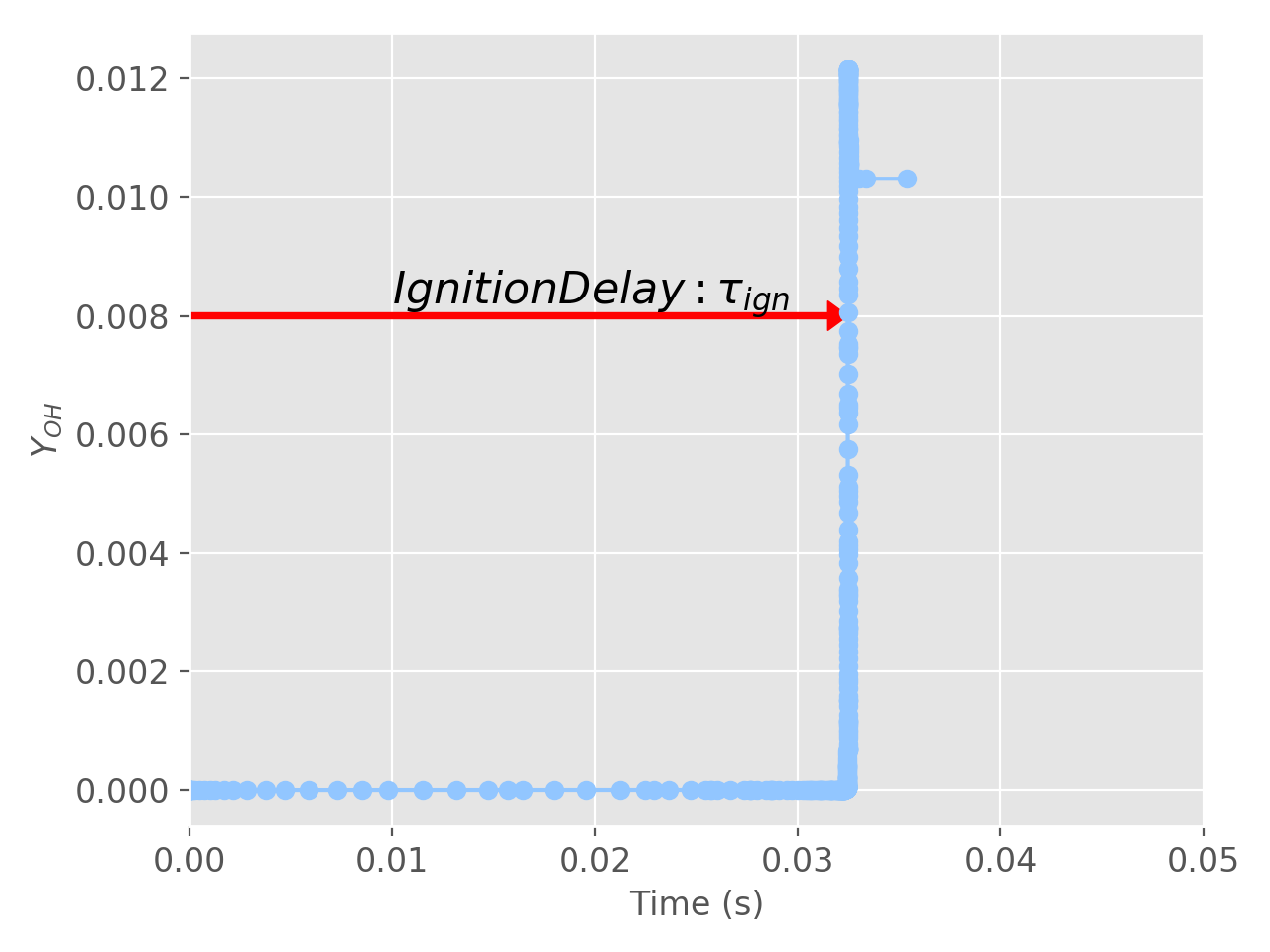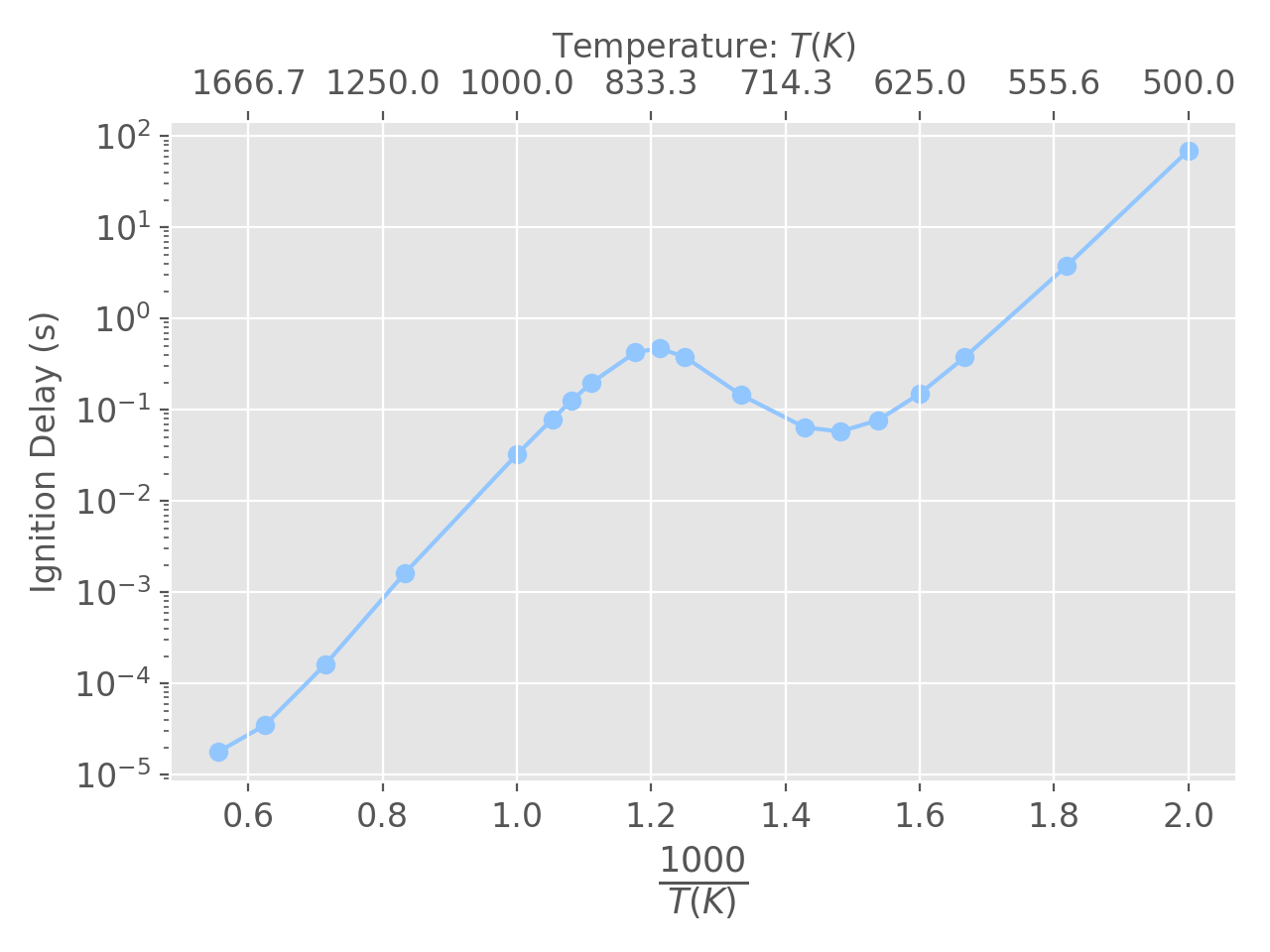# Batch Reactor Example¶

## Ignition delay computation¶

In this example we will illustrate how to setup and use a constant volume batch reactor. This reactor will then be used to compute the ignition delay of a gas at any temperature and pressure

The reactor (system) is simply an insulated box.

In :
from __future__ import division
from __future__ import print_function

import pandas as pd
import numpy as np

import time

import cantera as ct
print('Runnning Cantera version: ' + ct.__version__)

Runnning Cantera version: 2.5.0a2


### Import modules and set plotting defaults¶

In :
%matplotlib notebook
import matplotlib.pyplot as plt

plt.rcParams['axes.labelsize'] = 18
plt.rcParams['xtick.labelsize'] = 12
plt.rcParams['ytick.labelsize'] = 12
plt.rcParams['figure.autolayout'] = True

plt.style.use('ggplot')
plt.style.use('seaborn-pastel')


### Define the gas¶

In this example we will choose n-heptane as the gas. For a representative kinetic model, we use the 160 species mechanism by Seier et al. 2000, Proc. Comb. Inst.

In :
gas = ct.Solution('data/seiser.cti')


**** WARNING ****
For species c7h15o-1, discontinuity in h/RT detected at Tmid = 1391
Value computed using low-temperature polynomial:  21.8343
Value computed using high-temperature polynomial: 21.767


### Define reactor conditions : temperature, pressure, fuel, stoichiometry¶

In :
# Define the reactor temperature and pressure
reactorTemperature = 1000 #Kelvin
reactorPressure = 101325.0 #Pascals

gas.TP = reactorTemperature, reactorPressure

# Define the fuel, oxidizer and set the stoichiometry
gas.set_equivalence_ratio(phi=1.0, fuel='nc7h16', oxidizer={'o2':1.0, 'n2':3.76})

# Create a batch reactor object and add it to a reactor network
# In this example, the batch reactor will be the only reactor
# in the network
r = ct.IdealGasReactor(contents=gas, name='Batch Reactor')
reactorNetwork = ct.ReactorNet([r])

# now compile a list of all variables for which we will store data
stateVariableNames = [r.component_name(item) for item in range(r.n_vars)]

# use the above list to create a DataFrame
timeHistory = pd.DataFrame(columns=stateVariableNames)


### Define useful functions¶

In :
def ignitionDelay(df, species):
"""
This function computes the ignition delay from the occurence of the
peak in species' concentration.
"""
return df[species].idxmax()

In :
#Tic
t0 = time.time()

# This is a starting estimate. If you do not get an ignition within this time, increase it
estimatedIgnitionDelayTime = 0.1
t = 0

counter = 1;
while(t < estimatedIgnitionDelayTime):
t = reactorNetwork.step()
if (counter%10 == 0):
# We will save only every 10th value. Otherwise, this takes too long
# Note that the species concentrations are mass fractions
timeHistory.loc[t] = reactorNetwork.get_state()
counter+=1

# We will use the 'oh' species to compute the ignition delay
tau = ignitionDelay(timeHistory, 'oh')

#Toc
t1 = time.time()

print('Computed Ignition Delay: {:.3e} seconds. Took {:3.2f}s to compute'.format(tau, t1-t0))

# If you want to save all the data - molefractions, temperature, pressure, etc
# uncomment the next line
# timeHistory.to_csv("time_history.csv")

Computed Ignition Delay: 3.248e-02 seconds. Took 1.31s to compute


## Plot the result¶

### Figure illustrating the definition of ignition delay¶

In :
plt.figure()
plt.plot(timeHistory.index, timeHistory['oh'],'-o')
plt.xlabel('Time (s)')
plt.ylabel('$Y_{OH}$')

plt.xlim([0,0.05])
plt.arrow(0, 0.008, tau, 0, width=0.0001, head_width=0.0005,
plt.annotate(r'$Ignition Delay: \tau_{ign}$', xy=(0,0), xytext=(0.01, 0.0082), fontsize=16);## Illustration : NTC behavior¶

A common benchmark for a reaction mechanism is its ability to reproduce the Negative Temperature Coefficient behavior. Intuitively, as the temperature of an explosive mixture increases, it should ignite faster. But, under certain conditions, we observe the opposite. This is referred to as NTC behavior. Reproducing experimentally observed NTC behavior is thus an important test for any mechanism. We will do this now by computing and visualizing the ignition delay for a wide range of temperatures

### Define the temperatures for which we will run the simulations¶

In :
# Make a list of all the temperatures we would like to run simulations at
T = [1800, 1600, 1400, 1200, 1000, 950, 925, 900, 850, 825, 800,
750, 700, 675, 650, 625, 600, 550, 500]

estimatedIgnitionDelayTimes = np.ones(len(T))

# Make time adjustments for the highest and lowest temperatures. This we do empirically
estimatedIgnitionDelayTimes[:6] = 6*[0.1]
estimatedIgnitionDelayTimes[-2:] = 10
estimatedIgnitionDelayTimes[-1] = 100

# Now create a dataFrame out of these
ignitionDelays = pd.DataFrame(data={'T': T})
ignitionDelays['ignDelay'] = np.nan


Now, what we will do is simply run the code above the plots for each temperature.

In :
for i, temperature in enumerate(T):
# Setup the gas and reactor
reactorTemperature = temperature
reactorPressure = 101325.0
gas.TP = reactorTemperature, reactorPressure
gas.set_equivalence_ratio(phi=1.0, fuel='nc7h16', oxidizer={'o2':1.0, 'n2':3.76})
r = ct.IdealGasReactor(contents=gas, name='Batch Reactor')
reactorNetwork = ct.ReactorNet([r])

# Create and empty data frame
timeHistory = pd.DataFrame(columns=timeHistory.columns)

t0 = time.time()

t = 0
counter = 0
while t < estimatedIgnitionDelayTimes[i]:
t = reactorNetwork.step()
if not counter % 20:
timeHistory.loc[t] = r.get_state()
counter += 1

tau = ignitionDelay(timeHistory, 'oh')
t1 = time.time()

print('Computed Ignition Delay: {:.3e} seconds for T={}K. Took {:3.2f}s to compute'.format(tau, temperature, t1-t0))

ignitionDelays.at[i, 'ignDelay'] = tau

Computed Ignition Delay: 1.788e-05 seconds for T=1800K. Took 0.90s to compute
Computed Ignition Delay: 3.511e-05 seconds for T=1600K. Took 0.89s to compute
Computed Ignition Delay: 1.612e-04 seconds for T=1400K. Took 0.84s to compute
Computed Ignition Delay: 1.630e-03 seconds for T=1200K. Took 1.00s to compute
Computed Ignition Delay: 3.248e-02 seconds for T=1000K. Took 1.02s to compute
Computed Ignition Delay: 7.909e-02 seconds for T=950K. Took 1.11s to compute
Computed Ignition Delay: 1.252e-01 seconds for T=925K. Took 1.16s to compute
Computed Ignition Delay: 1.983e-01 seconds for T=900K. Took 1.20s to compute
Computed Ignition Delay: 4.266e-01 seconds for T=850K. Took 1.25s to compute
Computed Ignition Delay: 4.726e-01 seconds for T=825K. Took 1.23s to compute
Computed Ignition Delay: 3.795e-01 seconds for T=800K. Took 1.27s to compute
Computed Ignition Delay: 1.462e-01 seconds for T=750K. Took 1.48s to compute
Computed Ignition Delay: 6.427e-02 seconds for T=700K. Took 1.57s to compute
Computed Ignition Delay: 5.791e-02 seconds for T=675K. Took 1.57s to compute
Computed Ignition Delay: 7.723e-02 seconds for T=650K. Took 1.71s to compute
Computed Ignition Delay: 1.503e-01 seconds for T=625K. Took 1.79s to compute
Computed Ignition Delay: 3.754e-01 seconds for T=600K. Took 1.78s to compute
Computed Ignition Delay: 3.749e+00 seconds for T=550K. Took 2.20s to compute
Computed Ignition Delay: 6.945e+01 seconds for T=500K. Took 2.12s to compute


### Figure: ignition delay ($\tau$) vs. the inverse of temperature ($\frac{1000}{T}$).¶

In :
fig = plt.figure()
ax.set_xlabel(r'$\frac{1000}{T (K)}$', fontsize=18)
ax2.set_xlabel(r'Temperature: $T(K)$');